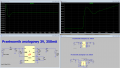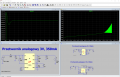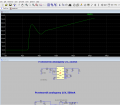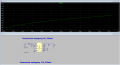# Problem with simulating in LTSpice

#### Xenon02

Joined Feb 24, 2021
489
Hello !

I've got a problem with simulating converters in LTSpice.
When I simulate 3 of my converters in one file their result is completelly different comparing with individual simulation :Here is an example where input is 7V and the output is 3V with I_max = 350mA. The left side is simulating individually and after 80us it has reached 3V but on the right side when I added another converter from 7V to 11V with I_max = 550mA then the result of this 3V with I_max = 350mA converter the values changed and after 80us I had only 48mV which I don't understand why is it like that, and it raises very slowly which is why I don't understand.

I don't want to change the LT3065 as well the rest of the converters but maybe there is any other way how to "fix it" ?

LTSpice simulations attached :

- Draft2<- contains all 3 converters
- Analogowy_1<- contains only one of 3 converters which is converter 3V, I_max = 350mA

Edit : I left the simulation for a while and this is the result :I don't know why it ends up like that.

#### Attachments

• 2 KB Views: 2
• 11.6 KB Views: 2
Last edited:

#### ronsimpson

Joined Oct 7, 2019
2,796
There are several things that might be the cause of this problem.
1) Normally I use a resistive load not a current source. There are very few current source loads in the real world. At start up it will try to get 350mA out of the PWM when it is at 0V.
2) Some time when I "run" the output will show 0V to 4V and the line at 3V. But some time it will show 2.99V to 3.01V and there is a ring. The voltage is actually the same but the difference is the scaling.

#### Xenon02

Joined Feb 24, 2021
489
1) Normally I use a resistive load not a current source. There are very few current source loads in the real world. At start up it will try to get 350mA out of the PWM when it is at 0V.
I have to use the current source as the load to force the maximum current to show that it works.

2) Some time when I "run" the output will show 0V to 4V and the line at 3V. But some time it will show 2.99V to 3.01V and there is a ring. The voltage is actually the same but the difference is the scaling.
I also thought that maybe it is about the scaling but looking at this :When I used the same converter just confuses me.
The rest converters works "ok" to the level that I reach required voltage like 1.8V or 11V, but 3V can't reach it's value when I add him in the file with the rest of my converters.

#### crutschow

Joined Mar 14, 2008
33,355
I have to use the current source as the load to force the maximum current to show that it works.
A current load is an infinite impedance which is never seen in the real world, and it may foul up the converters loop response.
Just use a small enough resistive load resistance to get the maximum current you want.

#### Xenon02

Joined Feb 24, 2021
489
A current load is an infinite impedance which is never seen in the real world, and it may foul up the converters loop response.
Just use a small enough resistive load resistance to get the maximum current you want.Edit :

I've used instead of 0.6 ohm I used 9.09 ohm
I guess it worked ?As I understand I cannot use to small resistor ? There is a R_min something like that ?

So if I understand it correctly, current source as a load makes the converter work incorrectly ? That why for one simulation for 80us I see a correct answer which is 3V but when I used current source the LTSpice calculates its behavior incorrectly and that's why I get the wrong answer which is 36mV?

Last edited:

#### boostbuck

Joined Oct 5, 2017
417
A 0.6 ohm load with 3 volts across it will require 5 amps from the supply. The chip will not be able to supply 5 amps so will be in current limit at startup.

If you wish to test 3V supply at 350mA then the load should be 8.6 ohms.

A current source is an inappropriate test load due to behaviours described above.

•Xenon02

#### crutschow

Joined Mar 14, 2008
33,355
As I understand I cannot use to small resistor ? There is a R_min something like that ?
From what source are you getting this info?

•Xenon02

#### Alec_t

Joined Sep 17, 2013
14,007
As I understand I cannot use to small resistor ? There is a R_min something like that ?
Spice won't let you use zero Ohms, but will handle a finite resistance.

•Xenon02